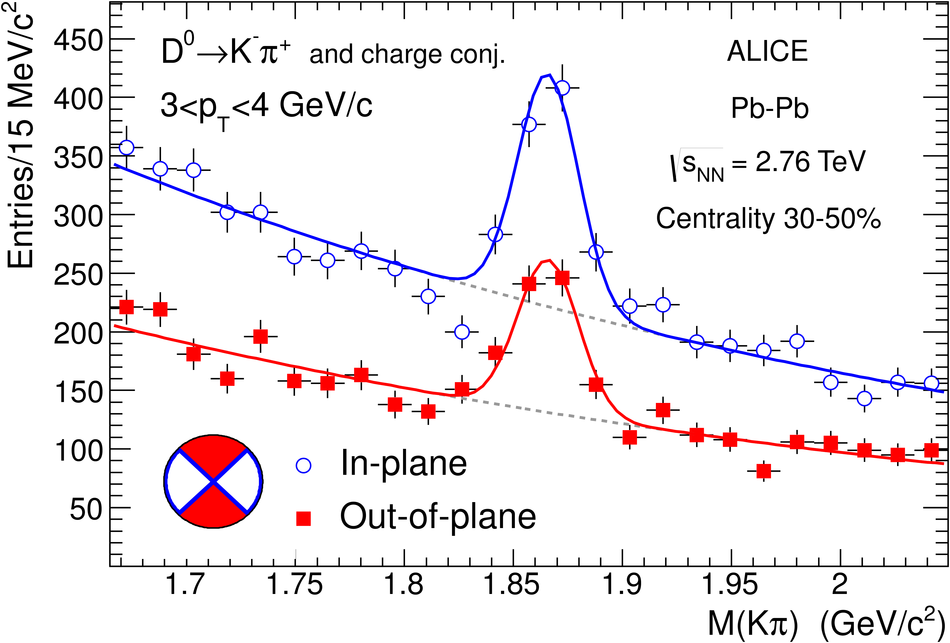# D meson elliptic flow in non-central Pb-Pb collisions at $\sqrt{s_{\rm NN}}$ = 2.76TeV

Azimuthally anisotropic distributions of D$^0$, D$^+$ and D$^{*+}$ mesons were studied in the central rapidity region ($|y|<0.8$) in Pb-Pb collisions at a centre-of-mass energy $\sqrt{s_{\rm NN}} = 2.76$ TeV per nucleon-nucleon collision, with the ALICE detector at the LHC. The second Fourier coefficient $v_2$ (commonly denoted elliptic flow) was measured in the centrality class 30-50% as a function of the D meson transverse momentum $p_{\rm T}$, in the range 2-16 GeV/$c$. The measured $v_2$ of D mesons is comparable in magnitude to that of light-flavour hadrons. It is positive in the range $2 < p_{\rm T} < 6$ GeV/$c$ with $5.7\sigma$ significance, based on the combination of statistical and systematic uncertainties.

Figures

## Figure 1

 Invariant mass distributions for $\Dzero$ candidates and their charge conjugates with $3< \pt< 4$ GeV/$c$ for $9.5\times10^6$ Pb-Pb collisions in the 30-50% centrality class. The distributions are shown separately for the in-plane (open symbols) and out-of-plane (closed symbols) intervals of azimuthal angle. The curves show the fit functions as described in the text.## Figure 2

 $v_{2}$ as a function of $\pt$ for prompt $\Dzero$, $\Dplus$ and $\Dstar$ mesons for Pb-Pb collisions in the centrality range 30-50%. The central value was obtained with the assumption $v_2^{\rm feed-down}=v_2^{\rm prompt}$. Vertical error bars represent the statistical uncertainty, empty boxes the systematic uncertainty due to the D meson anisotropy measurement and the event-plane resolution, and shaded boxes show the uncertainty from the contribution of D mesons from B feed-down.## Figure 3

 Average of $\Dzero$, $\Dplus$ and $\Dstar$ $v_2$ as a function of $\pt$, compared to charged-particle $v_2$ measured with the event plane (EP) method. The symbols are positioned horizontally at the average $\pt$ of the three D meson species.